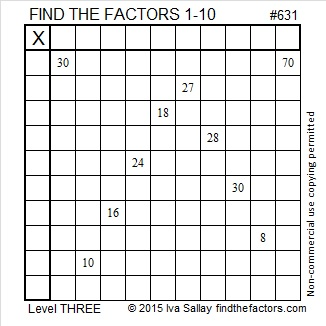# 631 and Level 3

631 is a prime number that happens to also be the sum of the eleven prime numbers from 37 to 79.

Sometimes mathematicians talk about triangular numbers, centered triangular numbers, hexagonal numbers, and centered hexagonal numbers. Is there any relationship between those terms?

• The sum of any three consecutive triangular numbers is a centered triangular number.
• ALL hexagonal numbers are triangular numbers.
• Most centered hexagonal numbers are NOT centered triangular numbers.

In my last post, I pointed out that 630 is a triangular number and a hexagonal number.

Well, amazingly the next number, 631, is a centered triangular number AND a centered hexagonal number.

How often does one more than a triangular number equal a centered triangular number? Also how often does one more than a hexagonal number equal a centered hexagonal number?

Let’s compare a couple of lists of these types of numbers up to 631. Red means a number is in both lists. Blue means the number in the 1st list is one less than the number in the 2nd list.

• Triangular numbers (with hexagonal numbers in bold type):
• 1, 3, 6, 10, 15, 21, 28, 36, 45, 55, 66, 78, 91, 105, 120, 136, 153, 171, 190, 210, 231, 253, 276, 300, 325, 351, 378, 406, 435, 465, 496, 528, 561, 595, 630.
• Centered triangular numbers (with numbers that are also centered hexagonal numbers in bold type):
• 1, 4, 10, 19, 31, 46, 64, 85, 109, 136, 166, 199, 235, 274, 316, 361, 409, 460, 514, 571, 631.

1 is the only number in the lists that is a triangular, centered triangular, hexagonal, and centered hexagonal number all at the same time. (1 is also a square number, a pentagonal number, etc.)

(630, 631) is the first combination of numbers that has BOTH a triangular number immediately followed by a centered triangular number AND a hexagonal number immediately followed by a centered hexagonal number.Print the puzzles or type the solution on this excel file: 10 Factors 2015-09-28

—————————————————————————————————

• 631 is a prime number.
• Prime factorization: 631 is prime.
• The exponent of prime number 631 is 1. Adding 1 to that exponent we get (1 + 1) = 2. Therefore 631 has exactly 2 factors.
• Factors of 631: 1, 631
• Factor pairs: 631 = 1 x 631
• 631 has no square factors that allow its square root to be simplified. √631 ≈ 25.11971.How do we know that 631 is a prime number? If 631 were not a prime number, then it would be divisible by at least one prime number less than or equal to √631 ≈ 25.1. Since 631 cannot be divided evenly by 2, 3, 5, 7, 11, 13, 17, 19, or 23, we know that 631 is a prime number.

—————————————————————————————————

A Logical Approach to solve a FIND THE FACTORS puzzle: Find the column or row with two clues and find their common factor. (None of the factors are greater than 10.)  Write the corresponding factors in the factor column (1st column) and factor row (top row).  Because this is a level three puzzle, you have now written a factor at the top of the factor column. Continue to work from the top of the factor column to the bottom, finding factors and filling in the factor column and the factor row one cell at a time as you go.This site uses Akismet to reduce spam. Learn how your comment data is processed.